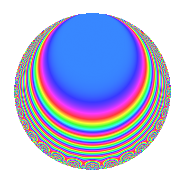# Properties

 Label 448.2.f.dLevel 448 Weight 2 Character orbit 448.f Analytic conductor 3.577 Analytic rank 0 Dimension 8 CM No Inner twists 4

# Related objects

## Newspace parameters

 Level: $$N$$ = $$448 = 2^{6} \cdot 7$$ Weight: $$k$$ = $$2$$ Character orbit: $$[\chi]$$ = 448.f (of order $$2$$ and degree $$1$$)

## Newform invariants

 Self dual: No Analytic conductor: $$3.57729801055$$ Analytic rank: $$0$$ Dimension: $$8$$ Coefficient field: $$\Q(\zeta_{16})$$ Coefficient ring: $$\Z[a_1, \ldots, a_{7}]$$ Coefficient ring index: $$2^{9}$$ Sato-Tate group: $\mathrm{SU}(2)[C_{2}]$

## $q$-expansion

Coefficients of the $$q$$-expansion are expressed in terms of a basis $$1,\beta_1,\ldots,\beta_{7}$$ for the coefficient ring described below. We also show the integral $$q$$-expansion of the trace form.

 $$f(q)$$ $$=$$ $$q -\beta_{2} q^{3} -\beta_{5} q^{5} -\beta_{4} q^{7} + ( 1 + \beta_{7} ) q^{9} +O(q^{10})$$ $$q -\beta_{2} q^{3} -\beta_{5} q^{5} -\beta_{4} q^{7} + ( 1 + \beta_{7} ) q^{9} + \beta_{1} q^{11} + ( -\beta_{3} - \beta_{5} ) q^{13} + ( \beta_{1} - \beta_{4} - \beta_{6} ) q^{15} + ( \beta_{3} - 2 \beta_{5} ) q^{17} + ( \beta_{2} + \beta_{4} - \beta_{6} ) q^{19} + ( \beta_{3} - \beta_{5} + \beta_{7} ) q^{21} + ( -3 \beta_{1} + 2 \beta_{4} + 2 \beta_{6} ) q^{23} + ( 1 + \beta_{7} ) q^{25} + ( -\beta_{4} + \beta_{6} ) q^{27} + ( 2 + 2 \beta_{7} ) q^{29} + ( 2 \beta_{2} + \beta_{4} - \beta_{6} ) q^{31} -\beta_{3} q^{33} + ( -3 \beta_{1} + \beta_{2} + \beta_{4} + \beta_{6} ) q^{35} + ( 2 - 2 \beta_{7} ) q^{37} + ( 3 \beta_{1} + \beta_{4} + \beta_{6} ) q^{39} + ( -2 \beta_{3} - 2 \beta_{5} ) q^{41} + ( -\beta_{1} + 2 \beta_{4} + 2 \beta_{6} ) q^{43} + ( \beta_{3} + \beta_{5} ) q^{45} + ( -2 \beta_{2} + \beta_{4} - \beta_{6} ) q^{47} + ( 1 + \beta_{3} - 2 \beta_{7} ) q^{49} + ( -4 \beta_{4} - 4 \beta_{6} ) q^{51} + 2 q^{53} + ( -\beta_{4} + \beta_{6} ) q^{55} + ( -4 - 3 \beta_{7} ) q^{57} + ( 3 \beta_{2} - \beta_{4} + \beta_{6} ) q^{59} + \beta_{5} q^{61} + ( -\beta_{1} - 2 \beta_{2} - \beta_{4} - 2 \beta_{6} ) q^{63} + ( -4 + 3 \beta_{7} ) q^{65} + ( 7 \beta_{1} - 2 \beta_{4} - 2 \beta_{6} ) q^{67} + ( -\beta_{3} + 4 \beta_{5} ) q^{69} + ( 4 \beta_{1} - \beta_{4} - \beta_{6} ) q^{71} + ( \beta_{3} + 4 \beta_{5} ) q^{73} + ( -3 \beta_{2} - \beta_{4} + \beta_{6} ) q^{75} + ( 2 + 2 \beta_{5} + \beta_{7} ) q^{77} + ( -6 \beta_{1} + \beta_{4} + \beta_{6} ) q^{79} + ( -3 - \beta_{7} ) q^{81} + ( -\beta_{2} + 2 \beta_{4} - 2 \beta_{6} ) q^{83} -8 q^{85} + ( -6 \beta_{2} - 2 \beta_{4} + 2 \beta_{6} ) q^{87} + \beta_{3} q^{89} + ( -5 \beta_{1} - 3 \beta_{2} + 2 \beta_{4} + 4 \beta_{6} ) q^{91} + ( -8 - 4 \beta_{7} ) q^{93} + ( 5 \beta_{1} - \beta_{4} - \beta_{6} ) q^{95} + ( -\beta_{3} + 6 \beta_{5} ) q^{97} + ( -\beta_{1} + 2 \beta_{4} + 2 \beta_{6} ) q^{99} +O(q^{100})$$ $$\operatorname{Tr}(f)(q)$$ $$=$$ $$8q + 8q^{9} + O(q^{10})$$ $$8q + 8q^{9} + 8q^{25} + 16q^{29} + 16q^{37} + 8q^{49} + 16q^{53} - 32q^{57} - 32q^{65} + 16q^{77} - 24q^{81} - 64q^{85} - 64q^{93} + O(q^{100})$$

Basis of coefficient ring:

 $$\beta_{0}$$ $$=$$ $$1$$ $$\beta_{1}$$ $$=$$ $$2 \zeta_{16}^{4}$$ $$\beta_{2}$$ $$=$$ $$-\zeta_{16}^{7} - \zeta_{16}^{5} + \zeta_{16}^{3} + \zeta_{16}$$ $$\beta_{3}$$ $$=$$ $$2 \zeta_{16}^{7} + 2 \zeta_{16}^{5} + 2 \zeta_{16}^{3} + 2 \zeta_{16}$$ $$\beta_{4}$$ $$=$$ $$-\zeta_{16}^{7} + \zeta_{16}^{6} + \zeta_{16}^{5} + \zeta_{16}^{4} - \zeta_{16}^{3} + \zeta_{16}^{2} + \zeta_{16}$$ $$\beta_{5}$$ $$=$$ $$\zeta_{16}^{7} - \zeta_{16}^{5} - \zeta_{16}^{3} + \zeta_{16}$$ $$\beta_{6}$$ $$=$$ $$\zeta_{16}^{7} + \zeta_{16}^{6} - \zeta_{16}^{5} + \zeta_{16}^{4} + \zeta_{16}^{3} + \zeta_{16}^{2} - \zeta_{16}$$ $$\beta_{7}$$ $$=$$ $$-2 \zeta_{16}^{6} + 2 \zeta_{16}^{2}$$
 $$1$$ $$=$$ $$\beta_0$$ $$\zeta_{16}$$ $$=$$ $$($$$$-\beta_{6} + 2 \beta_{5} + \beta_{4} + \beta_{3} + 2 \beta_{2}$$$$)/8$$ $$\zeta_{16}^{2}$$ $$=$$ $$($$$$\beta_{7} + \beta_{6} + \beta_{4} - \beta_{1}$$$$)/4$$ $$\zeta_{16}^{3}$$ $$=$$ $$($$$$\beta_{6} - 2 \beta_{5} - \beta_{4} + \beta_{3} + 2 \beta_{2}$$$$)/8$$ $$\zeta_{16}^{4}$$ $$=$$ $$\beta_{1}$$$$/2$$ $$\zeta_{16}^{5}$$ $$=$$ $$($$$$-\beta_{6} - 2 \beta_{5} + \beta_{4} + \beta_{3} - 2 \beta_{2}$$$$)/8$$ $$\zeta_{16}^{6}$$ $$=$$ $$($$$$-\beta_{7} + \beta_{6} + \beta_{4} - \beta_{1}$$$$)/4$$ $$\zeta_{16}^{7}$$ $$=$$ $$($$$$\beta_{6} + 2 \beta_{5} - \beta_{4} + \beta_{3} - 2 \beta_{2}$$$$)/8$$

## Character Values

We give the values of $$\chi$$ on generators for $$\left(\mathbb{Z}/448\mathbb{Z}\right)^\times$$.

 $$n$$ $$127$$ $$129$$ $$197$$ $$\chi(n)$$ $$-1$$ $$-1$$ $$1$$

## Embeddings

For each embedding $$\iota_m$$ of the coefficient field, the values $$\iota_m(a_n)$$ are shown below.

For more information on an embedded modular form you can click on its label.

Label $$\iota_m(\nu)$$ $$a_{2}$$ $$a_{3}$$ $$a_{4}$$ $$a_{5}$$ $$a_{6}$$ $$a_{7}$$ $$a_{8}$$ $$a_{9}$$ $$a_{10}$$
447.1
 0.923880 − 0.382683i 0.923880 + 0.382683i −0.382683 + 0.923880i −0.382683 − 0.923880i 0.382683 + 0.923880i 0.382683 − 0.923880i −0.923880 − 0.382683i −0.923880 + 0.382683i
0 −2.61313 0 1.08239i 0 −1.08239 + 2.41421i 0 3.82843 0
447.2 0 −2.61313 0 1.08239i 0 −1.08239 2.41421i 0 3.82843 0
447.3 0 −1.08239 0 2.61313i 0 2.61313 + 0.414214i 0 −1.82843 0
447.4 0 −1.08239 0 2.61313i 0 2.61313 0.414214i 0 −1.82843 0
447.5 0 1.08239 0 2.61313i 0 −2.61313 0.414214i 0 −1.82843 0
447.6 0 1.08239 0 2.61313i 0 −2.61313 + 0.414214i 0 −1.82843 0
447.7 0 2.61313 0 1.08239i 0 1.08239 2.41421i 0 3.82843 0
447.8 0 2.61313 0 1.08239i 0 1.08239 + 2.41421i 0 3.82843 0
 $$n$$: e.g. 2-40 or 990-1000 Embeddings: e.g. 1-3 or 447.8 Significant digits: Format: Complex embeddings Normalized embeddings Satake parameters Satake angles

## Inner twists

Char. orbit Parity Mult. Self Twist Proved
1.a Even 1 trivial yes
4.b Odd 1 yes
7.b Odd 1 yes
28.d Even 1 yes

## Hecke kernels

This newform can be constructed as the kernel of the linear operator $$T_{3}^{4} - 8 T_{3}^{2} + 8$$ acting on $$S_{2}^{\mathrm{new}}(448, [\chi])$$.